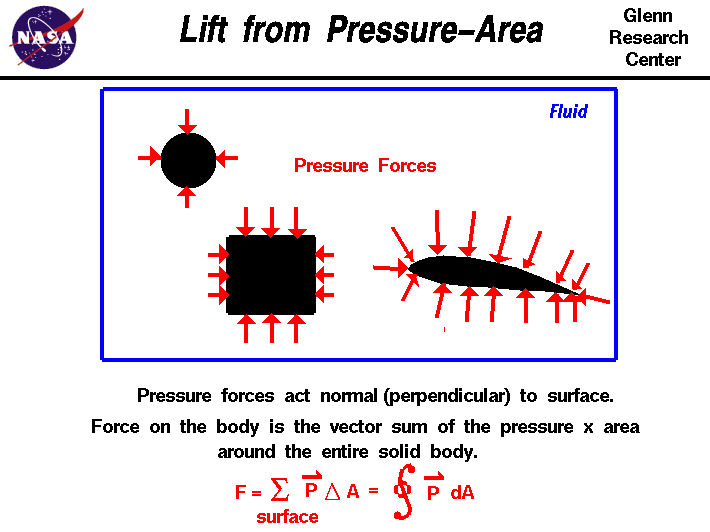+ Text Only Site
+ Non-Flash Version
+ Contact GlennWhen two solid objects interact in a mechanical process, forces are transmitted, or applied, at the point of contact. But when a solid object interacts with a fluid, things are more difficult to describe because the fluid can change its shape. For a solid body immersed in a fluid, the "point of contact" is every point on the surface of the body. The fluid can flow around the body and maintain physical contact at all points. The transmission, or application, of mechanical forces between a solid body and a fluid occurs at every point on the surface of the body. And the transmission occurs through the fluid pressure. Net Mechanical Force in A Static Fluid Pressure acts perpendicular to the surface of an object. To obtain the net mechanical force on the solid object, we have to multiply the pressure (perpendicular to the surface) times the area around the entire body. We also have to keep track of the direction of the pressure times the area. Consider each of the shapes discussed below: Square box. As shown in the figure, for a square box immersed in a static fluid, the pressure times the area on one side of the box is balanced by the pressure times the area on the other side, because the direction of the pressure force is different. The same is true for the top and bottom of the box. In a static fluid, there are no mechanical fluid forces on the surface of a box. (There would be buoyancy forces if the density of the solid were different from the fluid, but we are neglecting these effects for now and considering only the mechanical forces.) Cylinder. There are also no net mechanical forces on a cylinder in a static fluid. The pressure times area forces are all perpendicular to the surface and, therefore, pass through the center of the cylinder and cancel each other out. Airfoil. As for an airfoil in a static fluid, the pressure is a constant all around the airfoil. For the simple, closed surface around the airfoil, the normal (perpendicular) component of the pressure times the area will cancel out. In fact, for any simple closed contour, the normal component of the pressure times the area will sum to zero--no net force. Since the pressure is constant, we can also conclude that the sum of the normals to the surface, summed all around a simple closed surface, will be zero. Net Mechanical Force in A Moving Fluid BUT,... if the fluid is not static, but is in motion, the pressure varies around the closed surface. And the sum of the pressure (perpendicular to the surface) times the area around the body produces a net force. Since the fluid is in motion, we can define a flow direction along the motion. The component of the net force perpendicular to the flow direction is the lift. The component of the net force along the flow direction is the drag. These are definitions. In reality, there is only a single, net, integrated force caused by the pressure variations along a body. We can calculate the force on the body by using the equation at the bottom of the slide. We can break the surface up into a large number of small areas and sum up the pressure times the area around the whole surface. In the limit of infinitely small areas, this gives the integral of the pressure times the area around the closed surface. Pressure Variances in A Moving Fluid How does the pressure vary around a body if the fluid is in motion? In a fluid, the local pressure is related to the local velocity. If the velocity changes with location, the pressure changes as well. If the velocity is low, and no energy is added to the flow, the relation between pressure and velocity is given by Bernoulli's equation along a streamline. For an ideal fluid, with no boundary layers, the surface of an object is a streamline. If boundary layers are present, things are a little more confusing, since the external flow responds to the edge of the boundary layer. And the pressure on the surface is imposed from the edge of the boundary layer. If the boundary layer separates from the surface, it gets even more confusing. Summary So, to summarize, for any object immersed in a fluid, the mechanical forces are transmitted at every point on the surface of the body. The forces are transmitted through the pressure, which acts perpendicular to the surface. The net force can be found by integrating (or summing) the pressure times the area around the entire surface. For a moving flow, the pressure will vary from point to point because the velocity varies from point to point. For some simple flow problems, we can determine the pressure distribution (and the net force) if we know the velocity distribution by using Bernoulli's equation. But, how do we determine the velocity distribution around a body? Specifying the velocity is the source of error in two of the more popular incorrect theories of lift. What causes the velocity to change around a body? This leads us to an alternate way of looking at fluid forces involving the Navier-Stokes Equations . Activities: Guided Tours Navigation ..Beginner's Guide Home Page+ Inspector General Hotline + Equal Employment Opportunity Data Posted Pursuant to the No Fear Act + Budgets, Strategic Plans and Accountability Reports + Freedom of Information Act + The President's Management Agenda + NASA Privacy Statement, Disclaimer, and Accessibility CertificationEditor: Nancy Hall NASA Official: Nancy Hall Last Updated: May 07 2021 + Contact Glenn